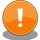# Integral Image FiltersThe content of this page has not been vetted since shifting away from MediaWiki. If you’d like to help, check out the how to help guide!Name Integral Image Filters Software Fiji Author Maintainer Stephan Saalfeld Source GitHub Status stable, active Category Plugins, Filtering

<div class="video"><iframe src="https://www.youtube.com/embed/p1mhZqj2VTY" width="400" height="300" frameborder="0" webkitallowfullscreen mozallowfullscreen allowfullscreen allow="accelerometer; encrypted-media; gyroscope; picture-in-picture"></iframe></div>

Integral images have been introduced in by Crow (1984)1 as a technique to improve texture rendering speed at multiple scales in perspective projections. The technique has since then been used for a number of applications. The most popular examples are fast normalized cross-correlation2, the Viola-Jones object detection framework3, and the Speeded Up Robust Feature (SURF) transform4. In Fiji, we currently use Integral Images for a number of basic statistic block filters.

## Basic Block Statistics with Integral Images (Summed-Area Tables)

### Mean

The mean $$\mu(X)$$ of a discrete set of random variables $$X=\{x_1,\dots,x_n\}$$ is defined as

$\mu=\sum_{i=1}^np_ix_i$

Let $$X$$ be the set of pixel values in a rectangular block with all pixel values having the same probability $$p_i=\frac{1}{n}$$, then

$\mu=\frac{1}{n}\sum_{i=1}^nx_i$

The sums can be generated from an Integral Image over $$I(\vec{x})$$ . For a two-dimensional image, the table can be generated in a single loop with, on average, 3~sums for calculation and 5~sums for data access per pixel. Using that table, the mean of an arbitrary rectangular block of pixels can be generated in constant time with 1 product and 3 sums for calculation and 2 products and 6 sums for data access.

### Variance

The variance $$\text{Var}(X)$$ of a discrete set of random variables $$X=\{x_1,\dots,x_n\}$$ is defined as

$\text{Var}(X) = \sum_{i=1}^np_i(x_i-\mu)^2 \quad\text{with}\quad \mu=\sum_{i=1}^np_ix_i$

Let $$X$$ be the set of pixel values in a rectangular block with all pixel values having the same probability $$p_i=\frac{1}{n}$$, then

$\text{Var}(X) = \frac{1}{n}\sum_{i=1}^n(x_i-\mu)^2 \quad\text{and}\quad \mu=\frac{1}{n}\sum_{i=1}^nx_i$

which expands to

$\text{Var}(x) = \frac{1}{n}\sum_{i=1}^n\left(x_i^2-2x_i\mu+\mu^2\right)$

$$ = \frac{1}{n}\sum_{i=1}^nx_i^2 - \frac{1}{n}\sum_{i=1}^n2x_i\mu + \mu^2$$

$ = \frac{1}{n}\sum_{i=1}^nx_i^2 - \frac{1}{n}\sum_{i=1}^n2x_i\mu + \frac{1}{n^2}\left(\sum_{i=1}^nx_i\right)^2$ $ = \frac{1}{n}\sum_{i=1}^nx_i^2 - \frac{2\mu}{n}\sum_{i=1}^nx_i + \frac{1}{n^2}\left(\sum_{i=1}^nx_i\right)^2$ $ = \frac{1}{n}\sum_{i=1}^nx_i^2 - \frac{2}{n^2}\left(\sum_{i=1}^nx_i\right)^2 + \frac{1}{n^2}\left(\sum_{i=1}^nx_i\right)^2$ $ = \frac{1}{n}\sum_{i=1}^nx_i^2 - \frac{1}{n^2}\left(\sum_{i=1}^nx_i\right)^2$ $ = \frac{1}{n}\sum_{i=1}^nx_i^2 - \left(\frac{1}{n}\sum_{i=1}^nx_i\right)^2$ $ = \frac{1}{n}\left(\sum_{i=1}^nx_i^2 - \frac{1}{n}\left(\sum_{i=1}^nx_i\right)^2\right)$

Both sums can be generated from two Integral Images over $$I(\vec{x})$$ and $$I(\vec{x})^2$$ respectively. For a two-dimensional image, both tables can be generated in a single loop with, on average, 1 product and 6 sums for calculation and 5 sums for data access per pixel. Using those, the variance of an arbitrary rectangular block of pixels can be generated in constant time with 3 products and 9 sums for calculation and 2 products and 6 sums for data access.

### Block Matching with Integral Images

We may deal with a situation where the intensities in two overlapping image regions $$X$$ and $$Y$$ might vary in brightness and contrast. Then, a simple estimator like e.g. the Mean Square Error (MSE) cannot be used as a similarity measure because it is not invariant with respect to a linear transformation. Instead, an appropriate measure for linear dependency would serve the purpose. The Pearson Product-Moment Correlation Coefficient (PMCC) $$\rho_{X,Y}$$ is an appropriate measure for linear dependency

$\rho_{XY} = \frac{\sigma_{XY}}{\sigma_{X}\sigma_{Y}}$

which, for $$X$$ and  being a finite sample with $$n$$~elements each gives the Correlation Coefficient $$r\_{XY}$$

$r_{XY} = \frac{\sum_{i=1}^n(x_i-\mu_X)(y_i-\mu_Y)}{\sqrt{\sum_{i=1}^n(x_i-\mu_X)^2}\sqrt{\sum_{i=1}^n(y_i-\mu_Y)^2}}\quad\text{with}\quad\mu_X = \frac{1}{n}\sum_{i=1}^nx_i$

that can be transformed yielding a set of independent sums. For the numerator, that is

$\sum_{i=1}^n(x_i-\mu_X)(y_i-\mu_Y) = \sum_{i=1}^nx_iy_i-\sum_{i=1}^nx_i\mu_Y-\sum_{i=1}^ny_i\mu_X+\sum_{i=1}^n\mu_X\mu_Y$ $= \sum_{i=1}^nx_iy_i-\mu_Y\sum_{i=1}^nx_i-\mu_X\sum_{i=1}^ny_i+n\mu_X\mu_Y$ $= \sum_{i=1}^nx_iy_i-\frac{1}{n}\sum_{i=1}^ny_i\sum_{i=1}^nx_i$

For the denominator, it is handy to multiply with $$\frac{n}{n}$$ first

$r_{XY} = \frac{\sum_{i=1}^nx_iy_i-\frac{1}{n}\sum_{i=1}^ny_i\sum_{i=1}^nx_i}{\sqrt{\sum_{i=1}^n(x_i-\mu_X)^2}\sqrt{\sum_{i=1}^n(y_i-\mu_Y)^2}}$ $= \frac{n\sum_{i=1}^nx_iy_i-\sum_{i=1}^ny_i\sum_{i=1}^nx_i}{n\sqrt{\sum_{i=1}^n(x_i-\mu_X)^2}\sqrt{\sum_{i=1}^n(y_i-\mu_Y)^2}}$ $= \frac{n\sum_{i=1}^nx_iy_i-\sum_{i=1}^ny_i\sum_{i=1}^nx_i}{\sqrt{n\sum_{i=1}^n(x_i-\mu_X)^2}\sqrt{n\sum_{i=1}^n(y_i-\mu_Y)^2}}$

because

$n\sum_{i=1}^n(x_i-\mu_X)^2 = n\sum_{i=1}^n(x_i^2-2x_i\mu_X+\mu_X^2)$ $= n\sum_{i=1}^nx_i^2 - 2n\mu_X\sum_{i=1}^nx_i + \left(\sum_{i=1}^nx_i\right)^2$ $= n\sum_{i=1}^nx_i^2 - 2\left(\sum_{i=1}^nx_i\right)^2 + \left(\sum_{i=1}^nx_i\right)^2$ $= n\sum_{i=1}^nx_i^2 - \left(\sum_{i=1}^nx_i\right)^2$

yielding

$r_{XY} = \frac{n\sum_{i=1}^nx_iy_i - \sum_{i=1}^nx_i\sum_{i=1}^ny_i}{\sqrt{n\sum_{i=1}^nx_i^2 - \left(\sum_{i=1}^nx_i\right)^2}\sqrt{n\sum_{i=1}^ny_i^2 - \left(\sum_{i=1}^ny_i\right)^2}}$

which means that we can calculate $$r_{XY}$$ for each block at a fix offset of two images from five summed-area tables at constant time. In some situations (e.g. finding an extremum), it is sufficient to estimate $$r_{XY}^2$$ and the sign of $$r_{XY}$$. Then, the calculation of the two square roots can be avoided

$r_{XY}^2 = \frac{a^2}{\left(n\sum_{i=1}^nx_i^2 - \left(\sum_{i=1}^nx_i\right)^2\right)\left(n\sum_{i=1}^ny_i^2 - \left(\sum_{i=1}^ny_i\right)^2\right)}$

with

$a = n\sum_{i=1}^nx_iy_i - \sum_{i=1}^nx_i\sum_{i=1}^ny_i\quad\text{and}\quad{}\sgn(r_{XY}) = \sgn(a)$

</div> </div>

1.Invalid citation arguments: {"fn"=>2, "content"=>"conference", "first"=>"J. P.", "last"=>"Lewis", "booktitle"=>"Vision Interface", "volume"=>"95", "pages"=>"120–123", "publisher"=>"Canadian Image Processing and Pattern Recognition Society", "title"=>"Fast template matching", "year"=>"1995"}